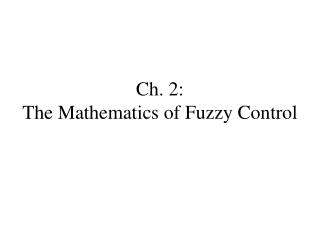DownloadDownload PresentationCh. 2: The Mathematics of Fuzzy Control

# Ch. 2: The Mathematics of Fuzzy Control

Télécharger la présentation## Ch. 2: The Mathematics of Fuzzy Control

- - - - - - - - - - - - - - - - - - - - - - - - - - - E N D - - - - - - - - - - - - - - - - - - - - - - - - - - -
##### Presentation Transcript

1. Ch. 2: The Mathematics of Fuzzy Control

2. The Mathematics of Fuzzy Control • Introduction: Fuzzy Sets • Fuzzy Relations • Approximate Reasoning • Representation of a Set of Rules

3. Introduction: Fuzzy Sets • Vagueness • Fuzzy Set Theory Versus Probability Theory • Classical Set Theory • Fuzzy Sets • Properties of Fuzzy Sets • Operations on Fuzzy Sets

4. How do we get computers to handle vagueness, like “Is person A tall?” What does it mean to be “tall”? Male/female? Geography/subpopulations? Canary Islanders as Spanish colonists thresholders estimators conservatives Vagueness Fuzzy logic grows out of the estimators approach to vagueness.

5. Fuzziness is due to lack of well defined boundaries. • Universe of discourse • precise membership degrees do not exist by themselves, but are only tendency indices that are subjectively assigned by an individual or a group of individuals. • Membership degree is an ordering. • Membership degrees are context dependent. • Fuzziness is not imprecision. We might agree on precisely how tall someone is, but disagree on the person’s tallness

6. Homework • Come up with 3 vague concepts, for each • define the universe of discourse for two different contexts

7. Fuzzy Set Theory vs Probability Fuzzy set theory is not probability theory. Enough said.

8. Classical Set Theory • Set operations • Intersection, Union, Complement, inference • and, or, not, if-then statements • Venn Diagrams • Logic • truth tables • Mathematization of Logic • Boolean algebra • characteristic function • Table 2.2, properties of classical set operations

9. Fuzzy Sets • Characteristic function to membership function • Expensive cars • natural numbers close to 6 • formulas vs /notation • Bell, S, Z membership functions • triangular (lambda), trapezoidal, S, Z membership functions (pg. 51)

10. Properties of fuzzy sets • Support • width • nucleus • height • convexity

11. Operations on Fuzzy Sets • Equality • inclusion • union • intersection • complement

12. Axiomatics (pg. 57) • Triangular norm (general intersection) • Archimedean property • Triangular co-norm (general union) • Complement • pp. 58-61 other norms and co-norms

13. Properties of Fuzzy Sets

14. Operations on Fuzzy sets

15. Fuzzy Relations • Classical Relations • Fuzzy Relations • Operations on Fuzzy Relations • The Extension Principle

16. Classical Relations

17. Fuzzy Relations

18. Operations on Fuzzy Relations

19. The extension principle

20. Approximate Reasoning • Introduction • Linguistic Variables • Fuzzy Propositions • Fuzzy If-Then statements • Inference Rules • The compositional Rule of Inference • Representing the Meaning of If-Then Rules

21. Intro to approx reasoning

22. Linguistic variables

23. Fuzzy Propositions

24. Fuzzy If-Then statements

25. Inference rules

26. The compositional Rule of Inference

27. Representing the Meaning of If-Then Rules

28. Representing a Set of Rules • Mamdani versus Godel • Properties of a Set of Rules • Completeness of a set of rules • Consistency of a set of rules • Continuity of a set of rules • Interaction of a set of rules

29. Mamdani vs Godel

30. Properties of a set of Rules

31. Completeness of a set of rules

32. Consistency of a set of rules

33. Continuity of a set of rules

34. Interaction of a set of rules

35. Chapter 2 Homework